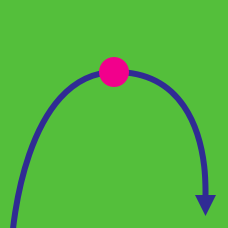Calculus

# Inflection Points

Given the function $f(x)=3x^3-9x^2+14x+36,$ what is the inflection point $(a,b)$ of $f(x)$?

If one of the inflection points of the curve $y = \ln (ax^2 + b)$ is $(1, \ln 50)$, what is the value of $a \times b$?

Let $f(x)=x^3+12x^2+3x-5.$ If $a$ and $b$ are constants such that the inflection point of the curve $y=f(x-a)+b$ is the origin $(0,0),$ what is the ordered pair $(a,b)?$

If $a$ and $b$ are constants such that the curve $f(x)=\ln (ax^2 + b)$ has an inflection point $(1,\ln 16),$ what is the value of $ab?$

The slope of the tangent line to the curve $y=ax^3-bx^2+cx$ at $x=2$ is $13,$ where $a, b$ and $c$ are constants. If $P=(1,7)$ is the inflection point of the curve, what is the value of $a+b+c$?

×